# Maximum entropy models

## Notation

•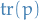for a probability distribution denotes the integral over all inputs if continuous, and sum if discrete

## Overview

• MaxEnt models are models which attempts to maximize the information (Shannon) entropy wrt. constraints on statistics (e.g. first moment) of the "data" or underlying generating distribution
• The optimization problem is then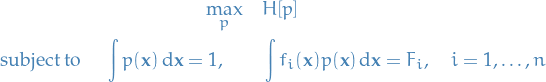where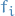are arbitrary functions on the input-space, andare the values which the expectation ofshould take

• Using Lagrange multipliers, we can write this as the minimization problem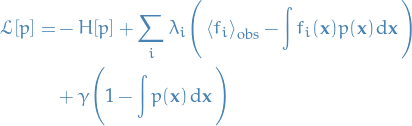where we've chosen these arbitraryto be the observed expectations of.

• Solving for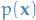by taking the functional derivative and setting to zero: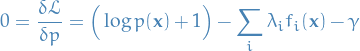The general form of the maximum entropy distribution is then given by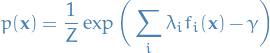whereFurthermore, the values of the Lagrange multipliers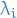are chosen to match the expectations of the functions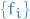, hence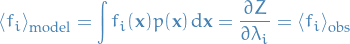i.e. the parameters of the distribution can be chosen s.t.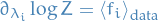Same holds for discrete random variables.

Still gotta estimate those moments yo!

## Principle of Maximum Entropy

### Shannon entropy

Say we want to define a functiondescribing the information, in terms of an eventwith probability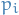; that is, how much information is acquired if we observe event?

Such a function one would expect to have the following properties:

1.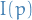is monotonoically decreasing in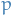, i.e. ifis large, then we gain little "information" by observing event.
2.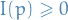, i.e. "information" is a non-negative quantity.
3.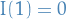, i.e. events occuring with a probability 1 provides no information.
4.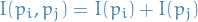for two independent eventsand, i.e. the "joint information" of two independent random variables is the information of both added together.

It turns out that the following equation satisfies exactly these properties (see here for how to deduce it):

The average amount of information received for an eventis given by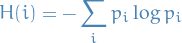### Justification

• Consider discrete probability distribution among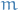mutually exclusive propositions
• Most informative distribution would occur when exactly one of the propositions was known to be true, in which case the information entropy would be zero (no "uncertainty").
• Least informative distribution would occur when all propositions are equally likely (uniform), in which case the information entropy would be equal to its maximum possible value: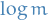.
• One can therefore view information entropy as a numerical measure of how uninformative a particular distribution is, ranging from zero to one.
• Choosing a distribution with maximum entropy we are in a way choosing the most uninformative distribution possible, and to choose a distribution with lower entropy would be to assume information which is unknown.

#### Walllis derivation - combinatorial argument

• https://en.wikipedia.org/wiki/Principle_of_maximum_entropy?oldformat=true#The_Wallis_derivation
• Makes no reference to entropy as a measure of "uncertainty"
• Want to make probability assignment tomutually exclusive propositions
• Let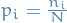be the probability of each of thepropositions, with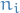being the number of times the i-th proposition "occurs"
• Probability of any particular results is the multinomial distribution: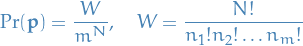• Most probably results it the one which maximizes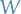, equivalently, we can maximize any monotonic increasing function of: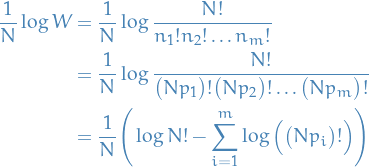• Assuming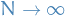we have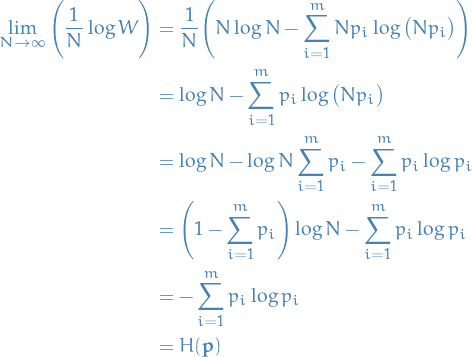### Issues

• Information about the probability distributions is intended to exist a priori
• Information provided by measurements is only an estimate of "true" information, hence we require methods for entropy estimation.

# Bibliography

• [paninski_2003] Paninski, Estimation of Entropy and Mutual Information, Neural Computation, 15(6), 1191–1253 (2003). link. doi.